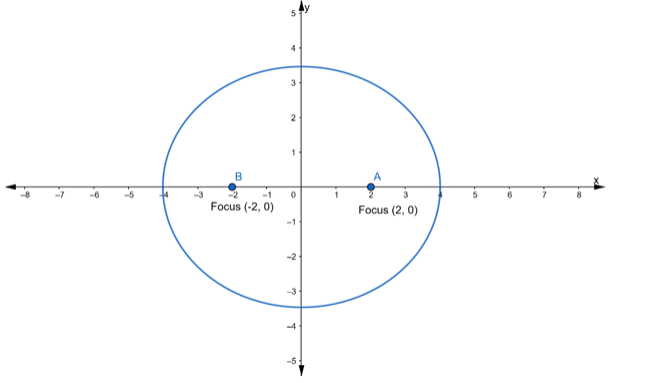Deepak Scored 45->99%ile with Bounce Back Crack Course. You can do it too!

# Find the equation of the ellipse whose foci are

Question:

Find the equation of the ellipse whose foci are (±2, 0) and the eccentricity is $\frac{1}{2}$

Solution:Let the equation of the required ellipse be

$\frac{x^{2}}{a^{2}}+\frac{y^{2}}{b^{2}}=1$

Given:

Coordinates of foci $=(\pm 2,0)$...(iii)

We know that,

Coordinates of foci $=(\pm c, 0)$...(iv)

$\therefore$ From eq. (iii) and (iv), we get

$c=2$

It is also given that

Eccentricity $=\frac{1}{2}$

we know that,

Eccentricity, e $=\frac{c}{a}$

$\Rightarrow \frac{1}{2}=\frac{2}{a}[\because c=2]$

$\Rightarrow a=4$

Now, we know that,

$c^{2}=a^{2}-b^{2}$

$\Rightarrow(2)^{2}=(4)^{2}-b^{2}$

$\Rightarrow 4=16-b^{2}$

$\Rightarrow b^{2}=16-4$

$\Rightarrow b^{2}=12$

Substituting the value of $a^{2}$ and $b^{2}$ in the equation of an ellipse, we get

$\frac{x^{2}}{a^{2}}+\frac{y^{2}}{b^{2}}=1$

$\Rightarrow \frac{x^{2}}{16}+\frac{y^{2}}{12}=1$